If Then Else Statement in Excel VBA (explained with examples) - EBOOKS AND TIPS FREE

# If Then Else Statement in Excel VBA (explained with examples)

In Excel VBA, IF Then Else statement allows you to check for a condition, and perform an action accordingly.
This is extremely valuable in many situations as we will see in the examples later in this tutorial.
To give you a simple example, suppose you have a list of grades in Excel and you want to highlight all those students who have scored an A. Now, if I ask you to do this manually, you will check each student’s grade and if it’s an A, you’ll highlight it, and if it isn’t, then you’ll leave it as is.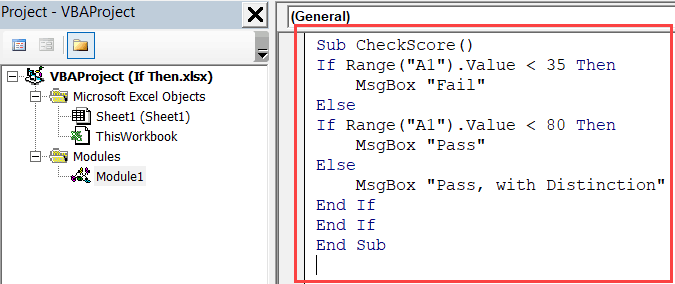The same logic can be built in VBA using the If Then Else statement as well (and of course do a lot more than just highlighting grades).
In this tutorial, I’ll show you different ways the ‘If Then Else’ construct can be used in Excel VBA, and some practical examples in action.
But before I get into the specifics, let me give you the syntax of the ‘IF Then Else’ statement.

## Syntax – IF Then Else

Below is the generic syntax of If Then Else construct in VBA
`IF condition Then true_code [Else false_code]`
Or
```IF condition Then
true_code
Else
false_code
End IF```
Note that the Else part of this statement is optional.
Now if you’re wondering what’s the difference between the two syntaxes, let me clarify.
The first syntax is a simple one-line IF THEN ELSE statement where you don’t need to use the END IF statement.
However, in the second syntax, the true_code part is in the second line. This is helpful when the code that you need to run in case the IF condition is true is long and consists of multiple lines.
When you split the IF statement into multiple lines, you need to tell VBA where the IF Then construct ends.
Hence you need to use the End IF statement.
In case you don’t use End IF when required, VBA will show you an error – “Block IF without END IF”

## Examples of Using IF Then Statement in VBA

To give you an idea of how the IF-THEN statement works in VBA, let me start with some basic examples (some practical and more useful examples are covered later in this tutorial).
Suppose you have a student’s score in cell A1 and you want to check whether the student passed the exam or not (passing marks threshold being 35).
Then you can use the following code:
```Sub CheckScore()
If Range("A1").Value >=35 Then MsgBox "Pass"
End Sub```
The above code has a single line of IF statement that checks the value in cell A1.
If it’s more than 35, it shows the message – “Pass”.
If it’s less than 35, nothing happens.
But what if you want to show a message in both the cases, whether a student passed or failed the exam.
The below code would do this:
```Sub CheckScore()
If Range("A1").Value >= 35 Then
MsgBox "Pass"
Else
MsgBox "Fail"
End If
End Sub```
The above code uses the IF as well as the ELSE statement to execute two different conditions. When the score is more than (or equal to) 35, the IF condition is true, and the code right below it gets executed (everything before the Else statement).
But when the IF condition is FALSE, the code jumps to the Else part and executes the code block in it.
Note that when we use a single line of IF Then statement, we don’t need to use End IF. But when we split it into more than one line, we need to use the End If statement.

## Nested IF Then (Multiple IF Then statements)

So far we have used a single IF Then statement.
In case you have multiple conditions to check, you can use:
• Multiple IF conditions
• If Then Else statement
• IF Then ElseIf Else construct
Let me show you how these differ and how to use this in Excel VBA.

### Multiple IF Then Statements

Let’s take the same example of using a student’s score.
If the student scores less than 35, the message to display is ‘Fail’, if the score is more than or equal to 35, the message to display is ‘Pass’.
We can use the below code to get this done:
```Sub CheckScore()
If Range("A1").Value < 35 Then MsgBox "Fail"
If Range("A1").Value >= 35 Then MsgBox "Pass"
End Sub```
You can use multiple IF Then statement as shown above. While this works, it’s not an example of good coding (as you will see the alternatives below).
In case you decide to use this, remember that these statements should either be independent or mutually exclusive. The important thing to know here is that in the above construct, all the IF statements are evaluated and the ones where the condition is true, the code is executed.
So even if the first IF statement is correct, the second would still be evaluated.

### IF Then Else Statement

Suppose this time, instead of just displaying the message Pass/Fail, we have one more condition.
If the student scores less than 35, the message to display is ‘Fail’, if the score is more than or equal to 35, the message to display is ‘Pass’, and if the score is more than 80, the message to display is ‘Pass, with Distinction’.
We can use the below code to get this done:
```Sub CheckScore()
If Range("A1").Value < 35 Then
MsgBox "Fail"
Else
If Range("A1").Value < 80 Then
MsgBox "Pass"
Else
MsgBox "Pass, with Distinction"
End If
End If
End Sub```
In the above code, we have used multiple IF statements (nested IF Then) with the help of Else.
So there is an ‘IF Then Else’ construct within an ‘IF Then Else’ construct. This type of nesting allows you to check for multiple conditions and run the relevant block of code.
IF Then ElseIf Else Statement
The above code (that we saw in the previous section) can be further optimized by using the ElseIf statement.
Here is what we’re trying to do – If the student scores less than 35, the message to display is ‘Fail’, if the score is more than or equal to 35, the message to display is ‘Pass’, and if the score is more than 80, the message to display is ‘Pass, with Distinction’.
```Sub CheckScore()
If Range("A1").Value < 35 Then
MsgBox "Fail"
ElseIf Range("A1").Value < 80 Then
MsgBox "Pass"
Else
MsgBox "Pass, with Distinction"
End If
End Sub```
The above code uses ElseIf, which allows us to keep all the conditions within one single IF Then statement.

## Using AND and OR in IF Then Else

So far in this tutorial, we have only checked for a single condition at a time.
However, when you have multiple dependent conditions, you can use the AND or OR statement with the IF conditions.
Below is the syntax of using AND/OR condition with the IF Then statement.
```IF Condition1 AND Condition2 Then
true_code
Else
false_code
End IF```
In the above code, only when both Condition1 and Condition2 are met, the true_code is executed. Even if one of the conditions is false, it will execute the false_code.
With OR, even if one of the conditions are true, it will execute the true_code. Only when all the conditions are false, it executes the false_code.
Now let’s see how AND and OR statement work with the IF Then Else construct.
Suppose you have the scores for two subjects instead of one, and you want to check for the following conditions:
• Fail – When the score is less than 35 in any of the subjects.
• Pass – When the score is more than or equal to 35, but less than 80 in both the subjects.
• Pass, with Distinction – When the score is more than 35 in both the subjects and is more than or equal to 80 in one or both the subjects.
Here is the code that will do this:
```Sub CheckScore()
If Range("A1").Value < 35 Or Range("B1").Value < 35 Then
MsgBox "Fail"
ElseIf Range("A1").Value < 80 And Range("B1").Value < 80 Then
MsgBox "Pass"
Else
MsgBox "Pass, with Distinction"
End If
End Sub```
The above code uses both OR and AND statements.
You can also write this same code with a slight change (using OR instead of AND).
```Sub CheckScore()
If Range("A1").Value < 35 Or Range("B1").Value < 35 Then
MsgBox "Fail"
ElseIf Range("A1").Value > 80 Or Range("B1").Value > 80 Then
MsgBox "Pass, with Distinction"
Else
MsgBox "Pass"
End If
End Sub```
Both the above VBA codes will give you the same result. Personally, I prefer the first one as it has a logical flow of checking the scores (but that’s just me).

## Using Not Equal to in If Then

In all the examples above, we have used the conditions that check whether a value equal to a specified value or not.
You can also use similar codes when checking when the value is not equal to a specified value in the VBA code. Not equal to represented by <> the Excel VBA.
To see a practical example of using <>, have a look at Example 1 below.

## Using If Then Else with Loops in VBA

So far, we have been going through some examples that are good to understand how the ‘IF-THEN’ statements work in VBA, however, are not useful in the practical world.
If I need to grade students, I can easily do that using Excel functions.
So let’s have a look at some useful and practical examples that can help you automate some stuff and be more efficient.

### Example 1 – Save and Close All Workbooks Except The Active Workbook

If you have a lot of workbooks open and you quickly want to close all, except the active workbook, you can use the below code,
```Sub SaveCloseAllWorkbooks()
Dim wb As Workbook
For Each wb In Workbooks
On error resume next
If wb.Name <> ActiveWorkbook.Name Then
wb.Save
wb.Close
End If
Next wb
End Sub```
The above code would save and close all the workbooks (except the active one).
It uses the For Next loop to go through the collection of all the open workbooks and checks the name using the IF condition.
If the name is not the same as that of the Active workbook, it saves and closes it.
In case there is a VBA code in any of the workbooks and you haven’t saved it as .xls or .xlsm, you will see a warning (as the vba codes are lost when you save it in .xlsx format).

### Example 2 – Highlight Cells with Negative Values

Suppose that you have a column full of numbers and you want to quickly highlight all the cells with negative values in red, you can do that using the below code.
```Sub HighlightNegativeCells()
Dim Cll As Range
For Each Cll In Selection
If Cll.Value < 0 Then
Cll.Interior.Color = vbRed
Cll.Font.Color = vbWhite
End If
Next Cll
End Sub```
The above code uses the For Each loop and checks each cell in the selection that you have made. If the cell has a value that is negative, it’s highlighted in red with white font color.

### Example 3 – Hide All the Worksheet Except the Current Worksheet

In case you want to quickly hide all the worksheets except the active one, you can use the below code:
```Sub HideAllExceptActiveSheet()
Dim ws As Worksheet
For Each ws In ThisWorkbook.Worksheets
If ws.Name <> ActiveSheet.Name Then ws.Visible = xlSheetHidden
Next ws
End Sub```
The above code uses the For Each loop to go through a collection of worksheets. It checks the name of each worksheet and hides it if it’s not the active worksheet.

### Example 4 – Extract the Numeric Part from an Alphanumeric String

If you have alphanumeric strings in cells and you want to extract the numeric part from it, you can do that using the below code:
```Function GetNumeric(CellRef As String)
Dim StringLength As Integer
StringLength = Len(CellRef)
For i = 1 To StringLength
If IsNumeric(Mid(CellRef, i, 1)) Then Result = Result & Mid(CellRef, i, 1)
Next i
GetNumeric = Result
End Function```
This code will create a custom function in Excel that can use within the worksheet (just like a regular function).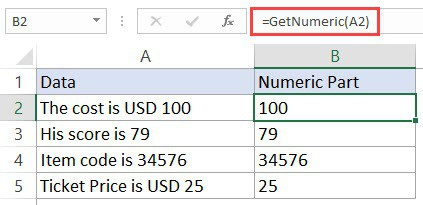## Where to Put the VBA Code?

Wondering where the VBA code goes in your Excel workbook?
Excel has a VBA backend called the VB editor. You need to copy and paste the code in the VB Editor module code window.
Here are the steps to do this:
1. Go to the Developer tab.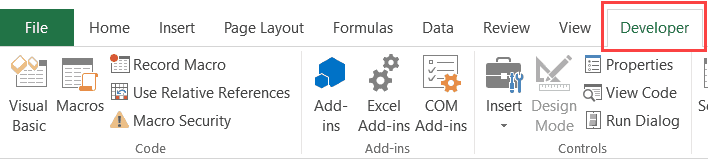2. Click on Visual Basic option. This will open the VB editor in the backend.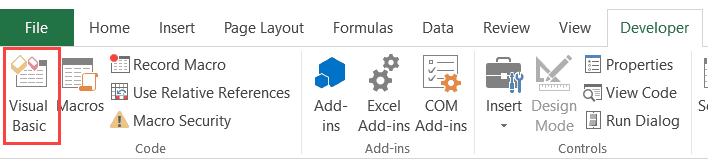3. In the Project Explorer pane in the VB Editor, right-click on any object for the workbook in which you want to insert the code. If you don’t see the Project Explorer go to the View tab and click on Project Explorer.
4. Go to Insert and click on Module. This will insert a module object for your workbook.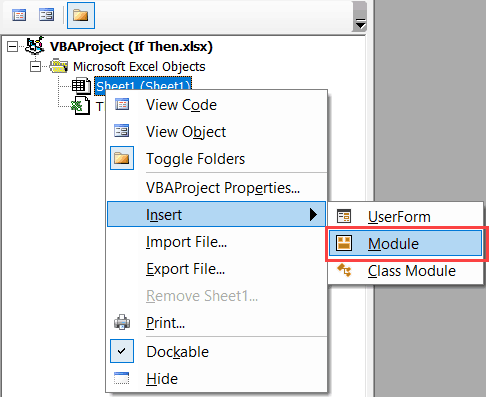5. Copy and paste the code in the module window.#evba #etipfree #eama #kingexcel